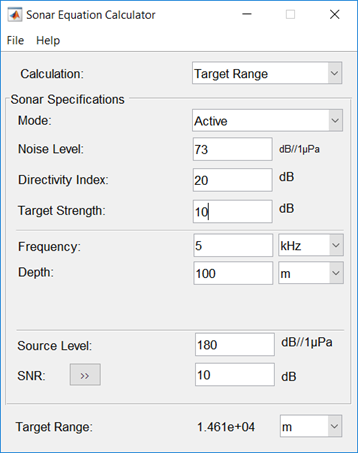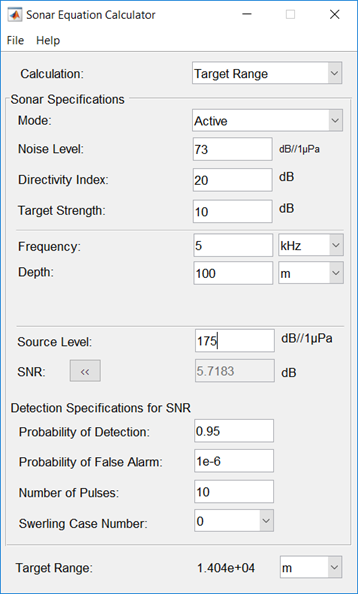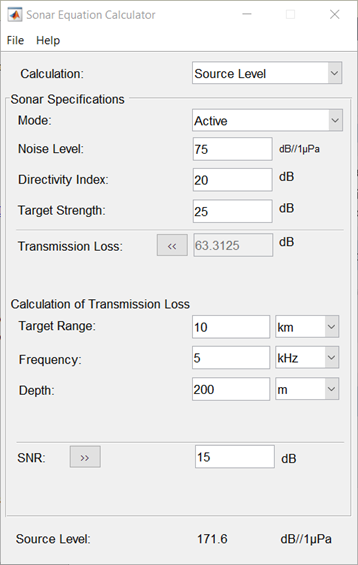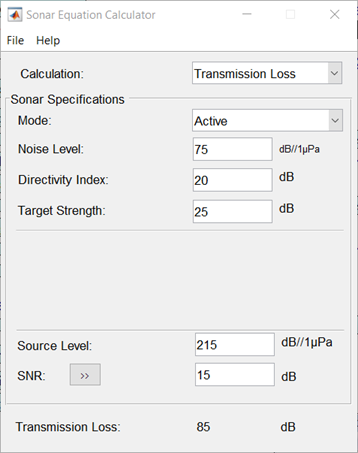Documentation

# Sonar Equation Calculator

Estimate maximum range, SNR, transmission loss and source level of a sonar system

## Description

The Sonar Equation Calculator app solves the basic sonar equation for monostatic sonar systems. The sonar equation relates transmission loss (or target range), source level, directivity, noise level, target strength, and signal SNR. You can solve for one of these quantities in terms of the others. Using this app, you can:

• Calculate the received SNR value from transmission loss (or equivalently, target range), source level, and noise level.

• Solve for transmission loss from sonar source level of the sonar, specified received SNR, and array directivity.

• Solve for target range from sonar source level of the sonar, specified received SNR, and array directivity.

• Calculate required source level from target range, source level, and received SNR.## Open the Sonar Equation Calculator App

• MATLAB® Toolstrip: On the Apps tab, under Signal Processing and Communications, click the app icon.

• MATLAB command line: Enter `sonarEquationCalculator`.

## Examples

### Maximum Detection Range of Active Sonar

Compute the maximum detection range of an active monostatic sonar designed to achieve an SNR of at least 10 dB. The operating frequency is 5 kHz, and the source level is 180 dB. Assume that the noise level is 73 dB, the receiver directivity is 20 dB, and the target strength is 10 dB.

• Set Calculation to ```Target Range```.

• Set Mode to `Active`.

• Set Noise Level to ` 73` dB//1μPa.

• Set receiver Directivity index to `20` dB.

• Set Target Strength to `10` dB.

• Set Frequency to `5` kHz.

• Set channel Depth to `100` m.

• Set Source Level to `180` dB//1μPa.

• Set required SNR to `10` dB.The maximum target range is 14.61 km.

### Maximum Detection Range for Multiple Pulses

Use multiple pulses to reduce the source level while maintaining the same maximum target range.

Start with the values set in the Maximum Detection Range of Active Sonar example.

• Click the arrows to the right of the SNR label to access the Detection Specifications for SNR options.

• Set Probability of Detection to `0.95`.

• Set Probability of False Alarm to `1e-6`.

• Set Number of Pulses to `10`.

• Reduce Source Level to `175`.

• Set the Swerling Case Number to `0` assuming a nonfluctuating target.The maximum detection range is 14.81 km, approximately the same as in the previous example, but the source level is reduced by 5 dB.

### Required Source Level for Monostatic Sonar

Compute the source level for an active monostatic sonar with a received SNR of 15 dB. The target range is 5 km and the target strength is 25 dB. Assume a 5-kHz sonar frequency.

• Set Calculation to ```Source Level```.

• Set Mode to `Active`.

• Set Noise Level to `75`.

• Set receiver Directivity index to `20` dB.

• Set Target Strength to `25`.

• Click the arrows to the right of the Transmission Loss label to access the Calculation of Transmission Loss options.

• Set the Range to `10.0` km.

• Set the Frequency to `5` kHz.

• Set the Depth to `200` m.

• Set SNR to `15` dB.The required source level is 171.6 dB//1 μPa.

### Received SNR for Monostatic Sonar

Compute the received SNR for a passive sonar with a source level of 140 db//1 μPa for a source 10.0 km away. Assume a 3-kHz sonar frequency.

• Set Calculation to `SNR`.

• Set Mode to `Passive`.

• Set Noise Level to `75`.

• Set receiver Directivity index to `20` dB.

• Click the arrows to the right of the Transmission Loss label to access the Calculation of Transmission Loss options.

• Set Range to `10.0` km.

• Set Frequency to `3` kHz.

• Set Depth to `200` m.

• Set Source Level to `140` dB//1 μPa.The received SNR is 23.16 dB.

### Transmission Loss of Monostatic Active Sonar

Compute the transmission loss for an active sonar that results in an SNR of 15 dB. The source level is 215 dB//1 μPa. Assume that the noise level is 75 dB//1 μPa, the receiver directivity is 20 dB, and the target strength is 10 dB.

• Set Calculation to ```Transmission Loss```.

• Set Mode to `Active`.

• Set Noise Level to `75` dB.

• Set receiver Directivity index to `20` dB.

• Set Target Strength to `25` dB.

• Set Source Level to `215` dB.

• Set required SNR to `15` dB.The transmission loss is 85 dB.

## Parameters

Select the type of calculation:

• `Target Range` -— solves for the maximum target range based on source level of the sonar and required received SNR.

• `Transmission Loss` -— computes the required transmit power from known target range and required received SNR.

• `Source Level` -— computes the source level from the range or transmission loss, and received SNR.

• `SNR` -— calculates the received SNR value based on known range and transmit power.

Specify whether the sonar is operating in active mode or passive mode. Active mode means that a signal is transmitted from a source, reflects off a target, and returns to the receiver which is collocated with the source. Active mode requires the specification of the reflector target strength. Passive mode means that the signal is transmitted from a source to a receiver along a direct path.

Noise level at sonar receiver, specified as a scalar. Units are dB//1μP.

Directivity index of receive array or element, specified as a scalar. Units are dB.

Target strength of reflector, specified as a scalar. Units are dB//1m2.

#### Dependencies

To enable this parameter, set the Mode parameter to `Active`.

Sound frequency, specified as a positive scalar. Default units are in kHz. You can also select `Hz`, `kHz`, or `MHz`.

Water channel depth. Default units are meters. You can select units in `m`, `km`, `mi`, or `nmi`.

Source level of sonar transmitter, specified as a scalar. Units are dB//1 μP.

Specify an SNR value, or calculate an SNR value using the Detection Specifications for SNR options. You can calculate the SNR required to achieve a particular probability of detection and probability of false alarm using the Shnidman equation. To calculate the SNR value:

1. Click the arrows to the right of the SNR label to access the Detection Specifications for SNR options.

2. Enter values for Probability of Detection, Probability of False Alarm, Number of Pulses, and Swerling Case Number.

#### Dependencies

To enable this parameter, set Calculation to `Target Range`, ```Transmission Loss```, or `Source Level`.

Specify the detection probability used to estimate SNR using the Shnidman equation.

#### Dependencies

To enable this parameter, set Calculation to `Target Range`, ```Transmission Loss```, or `Source Level`, and select the Detection Specifications for SNR options for the SNR parameter.

Specify the false alarm probability used to estimate SNR using the Shnidman equation.

#### Dependencies

To enable this parameter, set Calculation to `Target Range`, ```Transmission Loss```, or `Source Level`, and access the Detection Specifications for SNR options for the SNR parameter.

Specify the number of pulses. You can specify multiple pulses for noncoherent integration in the Shnidman equation.

#### Dependencies

To enable this parameter, set Calculation to `Target Range`, ```Transmission Loss```, or `Source Level`, and select the Detection Specifications for SNR options for the SNR parameter.

Specify the Swerling case number used to estimate SNR using the Shnidman equation. Swerling numbers characterize the detection problem for fluctuating pulses in terms of:

• a decorrelation model for the received pulses.

• the distribution of scatterers affecting the probability density function (pdf) of the target radar cross section (RCS).

The Swerling cases include two decorrelation models (scan-to-scan or pulse-to-pulse) and two radar cross section pdfs (based on the presence or absence of a dominant scatterer):

• `0` – Nonfluctuating pulses.

• `1` – Scan-to-scan decorrelation: Several randomly distributed scatterers with no dominant scatterer described by a Rayleigh/exponential PDF.

• `2` – Pulse-to-pulse decorrelation: Several randomly distributed scatterers with no dominant scatterer described by a Rayleigh/exponential PDF.

• `3` – Scan-to-scan decorrelation: Several scatterers with one dominant scatterer described by a chi-square PDF with 4 degrees of freedom.

• `4` – Pulse-to-pulse decorrelation: Several scatterers with one dominant scatterer described by a chi-square PDF with 4 degrees of freedom.

#### Dependencies

To enable this parameter, set Calculation to `Target Range`, ```Transmission Loss```, or `Source Level`, and select the Detection Specifications for SNR options for the SNR parameter.

Transmission loss in channel, specified as a scalar. Units are dB. For passive sonar modeling, specify a one-way transmission loss. For active sonar modeling, specify a two-way transmission loss. You can specify a transmission loss value, or calculate transmission loss using the Calculation of Transmission Loss options.

To calculate transmission loss:

1. Click the arrows to the right of the Transmission Loss label to access the Calculation of Transmission Loss menu.

2. Enter values for Target Range, Frequency, and Depth.

#### Dependencies

To enable this parameter, set Calculation to `Source Level` or `SNR`.

Target range, specified as a positive scalar. When Mode is `Passive`, target range is from source to receiver. When Mode is `Active`, target range is from source to reflecting target. Default units are in meters. You can also select `km`, `mi`, or `nmi`.

#### Dependencies

To enable this parameter, set the Calculation parameter to `Source Level` or `SNR` and click the arrow next to .

Signal frequency, specified as a positive scalar. Default units are in kHz. You can also select `Hz`, `kHz`, and `MHz`.

#### Dependencies

To enable this parameter, set Calculation to `Source Level` or `SNR` and click the arrow next to .

Channel depth, specified as a positive scalar. Default units are in meters. You can also select `km`, `mi`, and `nmi`.

#### Dependencies

To enable this parameter, set the Calculation parameter to `Source Level` or `SNR` and click the arrow next to .